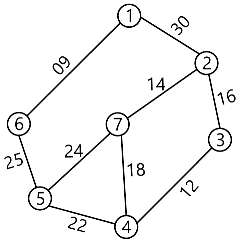MORE IN Analysis of Algorithms
MU Computer Engineering (Semester 4)
Analysis of Algorithms
May 2012
Total marks: --
Total time: --
INSTRUCTIONS
(1) Assume appropriate data and state your reasons
(2) Marks are given to the right of every question
(3) Draw neat diagrams wherever necessary

1 (a) Explain Big-oh, Omega and Theta Notations with the help of example. How do we analyse and measure time and space complexity of algorithms?
10 M
1 (b) Construct the Optimal Binary Search Tree for the identifier set(a1, a2, a3, a4) = (cout, float, if, while)
With P(1:4)=(1/2, 1/5, 1/10, 1/20) and
q(0:4) = (1/5, 1/10, 1/5, 1/20, 1/20)
10 M

2 (a) Explain Flow Shop Scheduling with the help of suitable examples.
10 M
2 (b) Write down Prims algorithm and solve the following problem:-10 M

3 (a) Write randomized quick sort algorithm and explain with the help of example.
10 M
3 (b) Explain 0/1 Knapsack using Branch and Bound method.
10 M

4 (a) Describe Travelling Salesperson problem. Explain how to solve using Branch and Bound.
10 M
4 (b) Write algorithm of sum of subsets. Solve following problem and draw portion of state space tree.
w=(5,7,10,12,15,18,20) and m=35.
Find all possible subsets of w that sum to m.
10 M

5 (a) Explain Strassen's Multiplication and derive its Time complexity.
10 M
5 (b) Write down Knuth-morris-Pratt Algorithm.
10 M

6 (a) Write down algorithm of job sequencing using deadlines. Solve the following Problem. N=5
(P1, P2, P3, P4, P5) = (20, 15, 10, 5, 1)
(d1, d2, d3, d4, d5) = (2, 2, 1 ,3, 3)
10 M
6 (b) Explain Hamiltonian Cycles Algorithm and draw static space tree.
10 M

Write short notes on (any four)
7 (a) Tries
5 M
7 (b) Randomized Algorithm
5 M
7 (c) N-Queens Problem
5 M
7 (d) Bellman and Ford Algorithm
5 M
7 (e) Optimal Storage on Tapes.
5 M

More question papers from Analysis of Algorithms